# Preface

This section presents basic solutions to the one dimensional heat equation on the finite interval [0,ℓ], subject to some boundary conditions. These solutions are obtained with the aid of the separation of variables method.

Introduction to Linear Algebra with Mathematica

Let us consider a heat conduction problem for a straight bar of uniform cross section and homogeneous material. With appropriate Cartesian coordinate system, the bar of length ℓ is oriented in the horizontal x-direction from x = 0 to x = ℓ. Suppose further that the sides of the bar are perfectly insulated so that no heat passes through them. We also assume that the cross-sectional dimensions are so small that the temperature u can be considered constant on any given cross section. Then u is a function of the axial coordinate x and the time t.

Graphics3D[Cylinder[{{0,0,0},{3,0,0}}, 1/3]]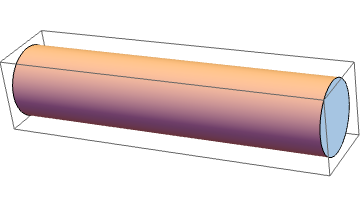The variation of temperature in the bar is governed by the partial differential equation, called the heat equation or diffusion equation:

\begin{equation} \label{EqBheat.1} \frac{\partial u}{\partial t} = \alpha\, \frac{\partial^2 u}{\partial x^2} \qquad \mbox{or for short} \qquad u_{t} = \alpha\, u_{xx} . \end{equation}
In general, a positive coefficient α>0, known as the thermal diffusivity, may depend on spatial variables, temperature, and pressure. However, we assume the thermal diffusivity to be constant in our problems, for simplicity. The parameter α depends only on the material from which the rod is made and is defined by
$\alpha = \frac{\kappa}{\rho\cdot c_p} ,$
where
Typical values of α are given in the following table.
Material Thermal diffusivity [m2/s] Thermal diffusivity [mm2/s]
Gold 1.27 × 10-4 127
Silver, pure (99,9%) 1.6563 × 10-4 165.63
Sandstone 1.12--1.19 × 10-6 1.15

In order to determine a unique solution to the heat transfer equation, we need to specify its initial temperature at t=0 and boundary conditions at end points. It is assumed that the initial temperature distribution in the bar is known

\begin{equation} \label{EqBheat.2} u(x,0) = f(x) , \end{equation}
where f is some given function. The physics of the problem dictates that this function must also satisfy some regularity conditions to match the temperature at the boundary. However, sometimes the corresponding initial boundary value problem (IBVP for short) can be considered without these regularity conditions with the expense of not uniform convergence of solution.

# Initial Boundary Value Problems for heat equations

The general solution of a partial differential equation is very sensitive to the boundary conditions. As such, the same equation may posses different properties under different sets of boundary conditions. In this section, we illustrate this fact by examining one dimensional heat conduction problems with different sets of boundary conditions. First, we consider a long metal bar of length ℓ which is much longer than it is thick. This means that we can treat the distribution of heat as a function of just one spatial variable x ∈ [0, ℓ] and time variable t. The bar is assumed to be insulated along its length so that it can only gain and lose heat through its ends. This means that the temperature distribution u(x, t) depends only on these two variables.

Keep in mind that, throughout this section, we will be solving the same one-dimensional homogeneous partial differential equation, Eq.\eqref{EqBheat.1}, which is called the diffusion equation (also known as the heat transfer equation). The heat conduction equation can now be paired up with a set of boundary conditions, of which we consider three most common types:

• The first type of boundary conditions or Dirichlet conditions (named after Peter Gustav Lejeune Dirichlet) when the temperature is prescribed at a boundary
$\left. u(x,t) \right\vert_{x\in \mbox{ boundary}} = T(t) .$
• The second type of boundary conditions or Neumann conditions ( named after Carl Neumann) when the heat flow (in general, the surface heat flux) is specified
$\left. \nabla u(x,t) \right\vert{x\in \mbox{ boundary}} = \left. \frac{\partial u(x,t)}{\partial x} \right\vert_{x\in \mbox{ boundary}} = q(t) .$
• The third type of boundary conditions or mixed boundary conditions usually include the following two cases.
• On one part of the boundary the temperature is specified (the Dirichlet conditions), while on another part the normal derivative is specified (Neumann conditions).
• When we observe convective heat transfer at the boundary
$\left( - \kappa\, \nabla \,u \right)_{x\in \mbox{ boundary}} = h\,A \left( u - T_{\infty} \right)_{x\in \mbox{ boundary}} ,$
where κ is the thermal conductivity of the material (with units W/(m K)), h is the convection heat transfer coefficient (with unites Watt/m² -K), and A is the surface area (units m²). The convection heat transfer coefficient is sometimes referred to as a film coefficient. It represents the thermal resistance of a relatively stagnant layer of fluid or air between a heat transfer surface and the fluid medium. It can also be defined through the Nusselt number:    $$\displaystyle h = \left( Nu \cdot \kappa \right) /L ,$$ where Nu is the Nusselt number, κ is the thermal conductivity, and L is the characteristic length.

Diffusion equation with the Dirichlet boundary condition

Consider a heat transfer problem for a thin straight bar (or wire) of uniform cross section and homogeneous material. Let the x-axis be chosen along the axis of the bar, and let x=0 and x=ℓ denote the ends of the bar. Suppose further that the lateral surface of the rod are perfectly insulated so that no heat transfers through them. We also assume that the cross-sectional dimensions are so small that the temperature u can be considered constant on any cross section. Then u is a function only of the axial coordinate x and the time t.

Assuming that no heat is being generated within the rod, the variation of temperature in it is governed by the partial differential equation (of parabolic type):

$\frac{\partial u}{\partial t} = \alpha \frac{\partial^2 u}{\partial x^2} \qquad\mbox{or for short} \qquad u_t = \alpha^2 u_{xx} , \qquad 0< x < \ell , \quad 0< t < t^{\ast} < \infty ,$
where α > 0 is a constant known as the thermal diffusivity. In addition, we assume that the ends x=0 and x=ℓ of the rod are held at fixed temperatures. More over, these temperatures are assumed to be zeroes, u(0,t) = 0℃ and u(ℓ,t) = 0℃ during all heat conduction process (for all t). This requirement is essential for the method of separation of variables that will be used to obtain the solution of Eq.\eqref{EqBheat.1}. In the following section, it will be shown how to bypass the homogeneity of the boundary conditions. For now, we consider the following boundary conditions:
$u(0,t) =0 , \qquad u(\ell, t) =0 , \quad t> 0.$
Besides the boundary conditions, we assume that we know the initial temperature distribution in the bar:
$u(x,0^{+}) = \lim_{t\downarrow 0} u(x,t) = f(x) , \qquad 0< x < \ell .$
Therefore, we get the initial boundary value problem (IBVP for short):
\begin{align*} &\mbox{Heat equation:} \qquad &u_t &= \alpha\,u_{xx} \qquad\mbox{for } 0 < x < \ell \mbox{ and } t > 0, \\ &\mbox{Boundary condition:} \qquad &u (0,t) &= 0 , \quad u (\ell , t) = 0, \qquad t > 0 , \\ &\mbox{Initial condition:} \qquad &u(x,0^{+}) &= \lim_{t\downarrow 0} u(x,t) = f(x) , \qquad 0 < x < \ell , \\ &\mbox{Regularity conditions:} \qquad &f(0) &= u (0,0) = 0 , \quad f(\ell ) = u (\ell , 0) = 0, \qquad \mbox{and} \qquad \lim_{t\to +\infty} u(x,t) = 0. \end{align*}
As previously stated, the regularity conditions are dictated by the physics of the problem. They consist of two parts: the first part requires the matching for the initial temperature with the homogeneous boundary conditions to maintain the continuity of the temperature distribution inside the rod. This condition can be disregarded because it does not affect the solvability of the IBVP. However, violation of this condition makes the given problem ill-posed and its numerical approximation may suffer instability or the Gibbs phenomena. The second condition is almost always satisfied because temperature approaches zero with time due to dissipation of energy.

The following animation gives the temperature distribution in a finite bar subject to homogeneous Dirichlet boundary conditions: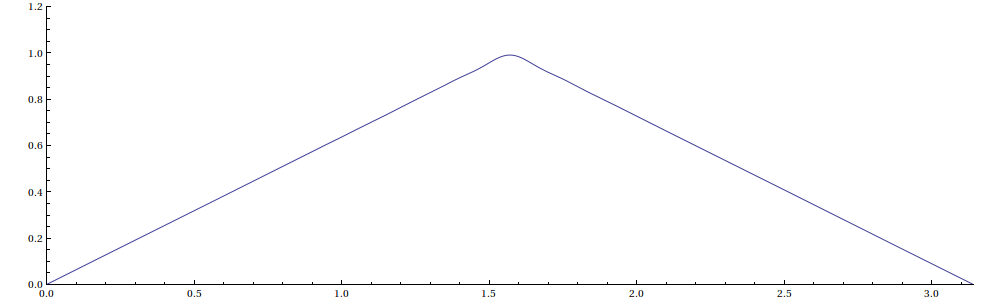The fundamental problem of heat conduction is to find u(x, t) that satisfies the heat equation and subject to the boundary and initial conditions. Under some light conditions on the initial function f, the formulated initial boundary value problem has a unique solution. This problem can be considered as a boundary value problem in xt-plane (see figure below). The solution u(x, t) of the heat equation is sought in the semi-infinite strip 0 < x < ℓ,    t > 0, subject to the requirement that u(x, t) must assume a prescribed values at each point on the boundary of this strip.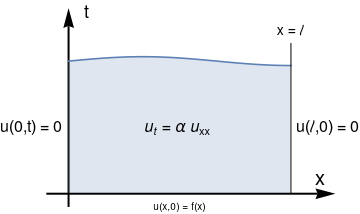Boundary value problem for the heat conduction equation. wave=Plot[3+0.1*Sin[0.5*t*Pi], {t,0,3}, Filling ->Axis, PlotRange-> {0,4}]; left = Graphics[ Text[Style["u(0,t) = 0", FontSize -> 16], {-0.5, 1.5}]]; right = Graphics[ Text[Style["u($ScriptL],0) = 0", FontSize -> 16], {3.5, 1.5}]]; ax = {Arrowheads[Large], Arrow[{{-0.3, 0}, {3.6, 0}}]} ; ax1 = Graphics[{Thick, Black, ax}] ; ay = {Arrowheads[Large], Arrow[{{0, -0.3}, {0, 4.2}}]} ; ay1 = Graphics[{Thick, Black, ay}]; txt1 = Graphics[Text[Style["x", FontSize -> 14], {3.4, 0.35}]]; txt2 = Graphics[ Text[Style["t", FontSize -> 14], {0.25, 4.1}]]; txt3 = Graphics[ Text[Style["x = \[ScriptL]", FontSize -> 14], {3, 3.7}]]; delta = Graphics[ Text[Style[ "\!$$\*SubscriptBox[\(u$$, $$t$$]\) = \[Alpha] \ \!$$\*SubscriptBox[\(u$$, $$xx$$]\) ", FontSize -> 16], {1.5, 1.5}]]; down = Graphics[ Text[Style[ "u(x,0) = f(x)"], {1.5, -0.3}]]; line = Graphics[ {{Black,Thick},Line[{{3,0},{3,3.4}}]}]; Show[ax1, ay1, wave, txt1, txt2, txt3, delta, left, right, down, line, AspectRatio -> 2/3] As it is seen from the figure, the boundary for the xt-domain is not smooth because it has two corner points (0,0) and (ℓ,0). In this case, we have to impose the regularity conditions to make the corresponding IBVP well-posed. Note that the equation and boundary conditions are homogeneous---only under these assumptions the separation of variables method is applicable. This gives us a way to seek its solution as superposition of some nontrivial solutions of the auxiliary problem: \[ u_t = \alpha\, u_{xx} \qquad (0< x < \ell) \quad u(0,t) = u(\ell ,t ) =0.$
So we seek nontrivial (which means not identically zero) solutions of the above auxiliary problem that are represented as a product of two functions:
$u(x,t) = X(x)\, T(t) ,$
where functions X = X(x) depends only on spatial variable x and T = T(t) depends on time variable t. Substituting this product into the heat equation, we get
$X(x)\,\dot{T}(t) = \alpha\, X''(x)\,T(t) \qquad \Longrightarrow \qquad \frac{\dot{T}}{\alpha^2 T(t)} = \frac{X'' (x)}{X(x)} ,$
in which the variables are separated. Since the left hand side depends only on time variable t, the right side depends on spatial variable x, and these variables are independent, these functions could be equal only when they are constant. Denoting this constant of separation as −λ, we arrive at two ordinary differential equations:
$\begin{split} \dot{T} + \alpha\, \lambda\,T(t) &= 0 , \\ X'' (x) + \lambda\,X(x) &= 0 . \end{split}$
The boundary condition at x=0 yields
$u(0,t) = X(0)\,T(t) =0 \qquad \Longrightarrow \qquad X(0) =0 ,$
otherwise we would get a trivial solution. From another boundary condition at x=ℓ, we have
$u(\ell ,t) = X(\ell )\,T(t) =0 \qquad \Longrightarrow \qquad X(\ell ) =0 ,$
Considering together the differential equation for X(x) and the homogeneous boundary conditions, we obtain the familiar Sturm--Liouville problem that we discussed previously:
$X'' (x) + \lambda\,X(x) = 0 , \qquad X(0) =0, \quad X(\ell ) =0 .$
This problem has nontrivial (not identically zero) solutions only for positive values of parameter λ of which we have a discrete number
$\lambda_n = \left( \frac{n\pi}{\ell} \right)^2 \qquad \Longrightarrow \qquad X_n = \sin \frac{n\pi x}{\ell} , \qquad n=1,2,\ldots .$
Therefore, Xn(x) are associated eigenfunctions that could be multiplied by any nonzero constant.

Now turning to differential equation for T and substituting $$n^2 \pi^2 /\ell^2$$ for λ, we have

$T' (t) + \alpha \left( \frac{n\pi}{\ell} \right)^2 T(t) =0 .$
Hence, T(t) = Tn(t) is proportional to the exponent $$\exp \left\{ - \frac{\alpha\, n^2 \pi^2 t}{\ell^2} \right\} , \ n=1,2,\ldots .$$ Multiplying functions Tn and Xn, we obtain for each n partial nontrivial solution of the heat equation satisfying the homogeneous boundary conditions of Dirichlet type:
$u_n (x,t) = X_n (x)\,T_n (t) = e^{- n^2 \pi^2 \alpha\, t / \ell^2} \,\sin \frac{n\pi x}{\ell} , \qquad n=1,2,\ldots .$
Each partial nontrivial solution un(x, t) satisfies the heat conduction equation \eqref{EqBheat.1} and the homogeneous boundary conditions. Obviously, an arbitrary linear combination of these functions will also satisfy the homogeneous heat equation and boundary conditions (in our case, they are of Dirichlet type). Now we apply the principle of superposition by seeking a solution to the given IBVP that is a linear combination of all partial nontrivial solutions
\begin{equation} \label{EqBheat.3} u(x,t) = \sum_{n\ge 1} c_n u_n (x,t) = \sum_{n\ge 1} c_n T_n (t)\, X_n (x) = \sum_{n\ge 1} c_n e^{- n^2 \pi^2 \alpha t / \ell^2} \,\sin \frac{n\pi x}{\ell} . \end{equation}
Assuming that the series \eqref{EqBheat.3} converges uniformely (this condition is required to interchange the order of limit and summation), the the sum function u(x, t) satisfies the partial differential equation $$u_t = \alpha\, u_{xx}$$ and homogeneous boundary conditions u(0,t) = 0 and u(ℓ,t) = 0 because the individual terms un have the same property. It remains only to satisfy the initial condition
\begin{equation} \label{EqBheat.4} u(x,0^{+}) = \lim_{t\downarrow 0} \sum_{n\ge 1} c_n u_n (x,t) = \sum_{n\ge 1} c_n u_n (x,0^{+}) = \sum_{n\ge 1} c_n \,\sin \frac{n\pi x}{\ell} = f(x) , \qquad 0< x < \ell . \end{equation}
So if we can move the limit under the sign of summation (this is possible when the series converges uniformly), then what is remained to do is to determine the coefficients cn so that the identity
$\sum_{n\ge 1} c_n \,\sin \frac{n\pi x}{\ell} = f(x) , \qquad 0< x < \ell ,$
holds In other words, we have to determine coefficients cn so that the Fourier sine series converges to the initial temperature distribution f(x) for 0<x<ℓ. According to Euler--Fourier formula, we have
\begin{equation} \label{EqBheat.5} c_n = \frac{2}{\ell} \int_0^{\ell} f(x)\,\sin \frac{n\pi x}{\ell}\,{\text d}x , \qquad n=1,2,\ldots . \end{equation}

Justification of solution

All that remains is to investigate whether the Fourier sine series representation \eqref{EqBheat.3} of u(x, t) can satisfy the heat equation, ∂u/∂t = α ∂²u/∂x². To do that, we must differentiate the Fourier sine series that leads to justification of performing term-by-term differentiation. What is important for it is to remember that the heat equation must be verified in the open domain 0 < x <∞, 0 < t <∞.

The expression \eqref{EqBheat.3} for the temperature is not easy to analyze, but the negative exponential factor in each term of the series causes the series to converge if the coefficients \eqref{EqBheat.5} do not grow exponentially. If the initial temperature is represented by a function f(x) of bounded variation, then these coefficients decrease and the total series \eqref{EqBheat.3} converges quite rapidly for tt0 > 0. This tells us that we can differentiate term by term of u(x, t) any times in the open domain 0 < x <∞, 0 < t <∞. In particular, the series

\begin{align*} \frac{\partial u}{\partial t} &= \sum_{n\ge 1} c_n T' (t)\, X_n (x) = \alpha \sum_{n\ge 1} c_n \left( \frac{n\pi x}{\ell} \right)^2 e^{- n^2 \pi^2 \alpha t / \ell^2} \,\sin \frac{n\pi x}{\ell} , \\ \frac{\partial^2 u}{\partial x^2} &= \sum_{n\ge 1} c_n T (t)\, X''_n (x) = - \sum_{n\ge 1} c_n \left( \frac{n\pi x}{\ell} \right)^2 e^{- n^2 \pi^2 \alpha t / \ell^2} \,\sin \frac{n\pi x}{\ell} . \end{align*}
converge in the open domain.

The solution series \eqref{EqBheat.3} converges actually in the semi-open domain 0 ≤ x <∞, 0 < t <∞. So the boundary conditions are also satisfies because the following relations hold

$\lim_{x \to 0} \sum_{n\ge 1} c_n T (t)\, X_n (x) = \sum_{n\ge 1} c_n T (t)\, \lim_{x \to 0} \, X_n (x) , \qquad \lim_{x \to \ell} \sum_{n\ge 1} c_n T (t)\, X_n (x) = \sum_{n\ge 1} c_n T (t)\, \lim_{x \to \ell} \, X_n (x) .$

It remains to show that the initial condition is satisfied. Its justification is based on validity of

$\lim_{t \to 0} \sum_{n\ge 1} c_n T (t)\, X_n (x) = \sum_{n\ge 1} c_n \lim_{t \to 0} T (t)\, X_n (x) = \sum_{n\ge 1} c_n T (0)\, X_n (x) = \sum_{n\ge 1} c_n X_n (x) = f(x).$
For small t, the series solution \eqref{EqBheat.3} may converge, but not uniformly, and proving the validity of the initial condition can be challengeable.

This means that the truncated series

$v_N (x,t) = \sum_{n=1}^N X_n (x) \,T_n (t) = \sum_{n=1}^N u_n (x,t)$
provides a good approximation to the true solution in the domain tt0 > 0 because every term un(x, t) satisfies exactly the heat conduction equation and the boundary conditions, and the the exponential tail of the remainder can be ignored. Therefore, the truncated series can be used for actual approximation to the solution for the moderate values of t, but it loses its fast convergence for small t, which requires additional attention.

If the initial temperature function f(x) is twice differentiable on the interval [0, ℓ] and f(0) = f(ℓ) = 0, then we can integrate by parts to obtain

$c_n = \frac{2}{\ell} \int_0^{\ell} f(x)\,\sin \frac{n\pi x}{\ell} \, {\text d} x = - \frac{2\ell}{n^2 \pi^2} \int_0^{\ell} f''(x)\,\sin \frac{n\pi x}{\ell} \, {\text d} x .$
This relation shows that the coefficients cn decay as n−2. So the series $$\sum_n c_n X_n (x)$$ converges uniformly for x ∈ [0, ℓ]. Therefore, we can interchange the limit as t → 0 and summation.

Example 1A: Consider a uniform rod of length ℓ = 3 meters long, insulated on sides, with thermal diffusivity 9. If initially the rod has a constant temperature of 20℃, but homogeneous Dirichlet boundary conditions are maintained, we come to the following IBVP:

\begin{equation*} \begin{split} &\text{(PDE)} \quad u_{t} = 9\, u_{xx} , \quad 0<x<3, \ 0< t\le t^{\ast} < \infty , \\ &\text{(BC)} \hspace{6.2mm} u(0,t) =0 , \quad u (3 ,t) =0 , \quad 0< t\le t^{\ast} < \infty , \\ &\text{(IC)} \qquad u(x,0^{+}) = 20 . \end{split} \end{equation*}
The temperature in the rod satisfies the heat conduction equation \eqref{EqBheat.1} with ℓ = 3 and initial temperature f(x) = 20 for 0 < x < 3. Thus, from Eq.\eqref{EqBheat.3}, the solution is
$u(x,t) = \sum_{n\ge 1} c_n e^{-n^2 \pi^2 t} \sin \frac{n\pi x}{3} ,$
where
$c_n = \frac{2}{3} \int_0^3 20\, \sin \frac{n\pi x}{3}\,{\text d} x = \frac{40}{n\pi} \left( 1 - \cos n\pi \right) = \frac{40}{n\pi} \times \begin{cases} 2, & \ \mbox{ for odd indices, } n= 2k+1 , \\ 0, & \ \mbox{ for even indices, } n= 2k. \end{cases}$
Mathematica helps:
40/3*Integrate[Sin[n*Pi*x/3], {x, 0, 3}]
(40 (3 - 3 Cos[n $Pi]]))/(3 n \[Pi]) Finally, we obtain \[ u(x,t) = \frac{80}{\pi} \sum_{k \ge 0} \frac{1}{2k+1} \,e^{-(2k+1)^2 \pi^2 t} \,\sin \frac{\pi \left( 2k+1 \right) x}{3} .$
The series solution converges rapidly in the domain tt0 > 0, but the initial conditions are difficult to verify because the series does not converge uniformly for small t and we cannot set t = 0 under the sign of summation because it converges conditionally.

Now we demonstrate how this problem can be solved with Mathematica. Firs, we define the heat partial differential equation that represents the temperature in the bar:

heatEq = D[u[x, t], t] == 9 * D[u[x, t], {x, 2}]
$$u^{(0,1)}[x, t] = 9\,u^{(2,0)}[x, t]$$
Then we solve the heat equation with the appropriate boundary conditions
heatEqSoln = u[x, t] /. DSolve[{heatEq, u[0, t] == 0, u[3, t] == 0, u[x, 0] == 20}, u[x, t], {x, t}][[1, 1]] /. K -> n
which gives exactly the same formula as before.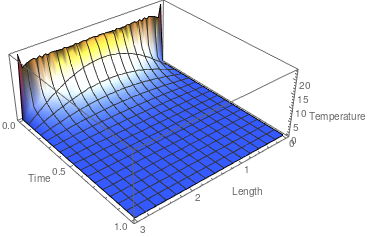We plot the temperature distribution inside the bar. Plot3D[heatEqSoln30[x, t], {x, 0, 3}, {t, 0, 1} , ColorFunction -> "TemperatureMap" , PlotRange -> All , AxesLabel -> {"Length", "Time", "Temperature"}] Temperature distribution. Mathematica code

As it is seen from the graph, there are ripples near the edge t = 0, which indicates poor convergence of the series. This is a confirmation that the given initial boundary value problem is ill-posed; so the corresponding numerical calculations must be done with care. The Gibbs phenomenon becomes clear when plotting the graph from a different perspective.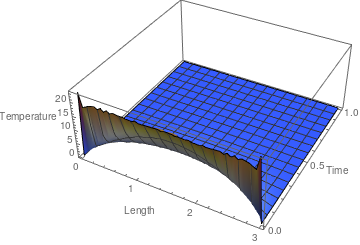The graph reveals a Gibbs phenomena near the edge t = 0. We take the truncated sum with 31 terms and plot it. u30[x_, t_] = 80/Pi*Sum[ Exp[-(2*k + 1)^2*Pi^2*t]*Sin[Pi*(2*k + 1)*x/3]/(2*k + 1), {k, 0, 30}]; Plot3D[u30[x, t], {x, 0, 3}, {t, 0, 1} , ColorFunction -> "TemperatureMap" , PlotRange -> All , AxesLabel -> {"Length", "Time", "Temperature"}] Temperature distribution. Mathematica code

In order to eliminate the Gibbs phenomena, we use the Cesàro summation:
$C_{30}(x,t) = \frac{80}{\pi} \sum_{k = 0}^{30} \left( 1 - \frac{k}{31} \right) \frac{1}{2k+1} \,e^{-(2k+1)^2 \pi^2 t} \,\sin \frac{\pi \left( 2k+1 \right) x}{3} .$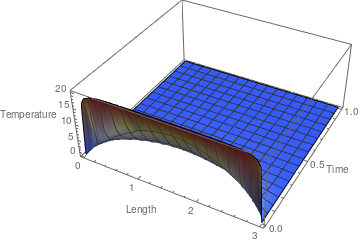The graph reveals a Gibbs phenomena near the edge t = 0. We take the truncated sum with 31 terms and plot it. C30[x_, t_] = 80/Pi*Sum[(1 - k/31)* Exp[-(2*k + 1)^2*Pi^2*t]*Sin[Pi*(2*k + 1)*x/3]/(2*k + 1), {k, 0, 30}]; Plot3D[C30[x, t], {x, 0, 3}, {t, 0, 1} , ColorFunction -> "TemperatureMap" , PlotRange -> All , AxesLabel -> {"Length", "Time", "Temperature"}] Cesàro summation for temperature approximation. Mathematica code

We plot temperature as a function of the position across the bar at different time values. First, we compare truncated versions of regular Fourier approximation and its Cesàro counterpart for tiny t = 0.001.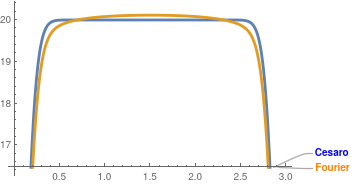We put two temperature profiles at t = 0.001 together for comparison, Plot[{Callout[u30[x, 0.001], "Fourier", LabelStyle -> {Bold, Orange}], Callout[C30[x, 0.001], "Cesaro", LabelStyle -> {Bold, Blue}]}, {x, 0, 3}, PlotStyle -> Thickness[0.01]] Fourier and Cesàro approximations. Mathematica code

Starting with t = 0.01, there is no visible difference in regular Fourier summation and corresponding Cesàro approximation.
Plot[u30[x, 0.1], {x, 0, 3}, PlotStyle -> Thickness[0.01]]
Plot[u30[x, 0.2], {x, 0, 3}, PlotStyle -> Thickness[0.01]]
Plot[u30[x, 2.1], {x, 0, 3}, PlotStyle -> Thickness[0.01]]
Of course, Mathematia complains that the exponential terms are too small for actual calculations. Therefore, 30-term approximation is not needed and first few terms is sufficient for accurate approximations.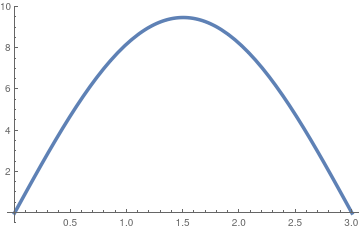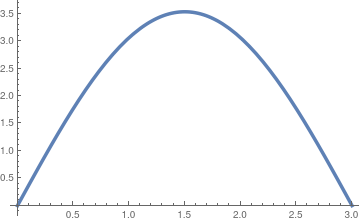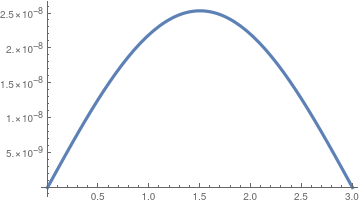Temperature distribution at t = 0.1. Temperature distribution at t = 0.2. Temperature distribution at t = 2.1.

In order plot the temperature as a function of the time at different position values along the bar, we compare its distribution with just first term
$s_0 (x,t) = \frac{80}{\pi}\, e^{-\pi^2 t} \sin \left( \frac{\pi x}{3} \right) .$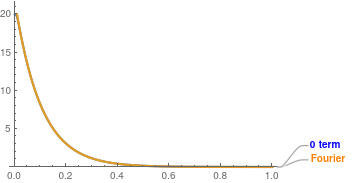We choose a typical point x = 2 and plot the truncated series with 30 terms along with its first term, s0(2, t). s0[x_, t_] = 80/Pi*Exp[-Pi^2*t]*Sin[Pi*x/3]; Plot[{Callout[u30[2, t], "Fourier", LabelStyle -> {Bold, Orange}], Callout[s0[2, t], "0 term", LabelStyle -> {Bold, Blue}]}, {t, 0, 1}, PlotStyle -> Thickness[0.008], PlotRange -> {0, 20}] Temperature distribution with time at x = 2. Mathematica code

If you looking at 1% accuracy (which is sufficient for plotting), then the figure shows that the single term s0(x, t) gives a good approximation.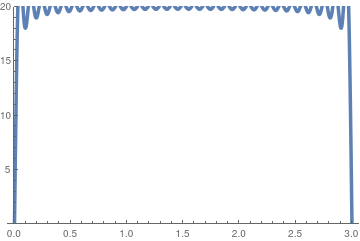Finally, we demonstrate annimation for temperature distribution with time. animation1 = Table[Plot[u30[x, t], {x, 0, 3}, PlotRange -> {0, 20}, PlotStyle -> Thickness[0.01] ], {t, 0, 0.5, 0.01}]; Export["1AAnimation.gif", animation1, "AnimationRepetitions" -> $Infinity]] ■ Example 1B: Consider conduction of heat in a rod 3 meters in length whose ends are maintained at 0℃. Initially, the rod was heated to 20℃ inside the interval 1 < x < 2. This yields the initial boundary value problem: \begin{equation*} \begin{split} &\text{(PDE)} \quad u_{t} = 9\, u_{xx} , \quad 0<x<3, \ 0< t\le t^{\ast} < \infty , \\ &\text{(BC)} \hspace{6.2mm} u(0,t) =0 , \quad u (3 ,t) =0 , \quad 0< t\le t^{\ast} < \infty , \\ &\text{(IC)} \qquad u(x,0^{+}) = H(x-1) - H(x-2) , \end{split} \end{equation*} where H(x) is the Heaviside function. Its solution, according to Eq.\eqref{EqBheat.3}, is \[ u(x,t) = \sum_{n\ge 1} c_n e^{-n^2 \pi^2 t} \sin \frac{n\pi x}{3} ,$
where
$c_n = \frac{2}{3} \int_1^2 \sin \frac{n\pi x}{3} \,{\text d} x = \frac{2}{n\pi} \left[ \cos \left( \frac{n\pi}{3} \right) - \cos \left( \frac{2n\pi}{3} \right) \right] , \qquad n=1,2,3,\ldots .$
2*Integrate[Sin[n*Pi*x/3], {x, 1, 2}]/3
(2 (Cos[(n $Pi])/3] - Cos[(2 n \[Pi])/3]))/(n \[Pi]) Or you may want to introduce the piecewise continuous function and then integrate f2[x_] = HeavisideTheta[x - 1] - HeavisideTheta[x - 2]; cn = 2/3*Integrate[f2[x]*Sin[n*Pi*x/3], {x, 0, 3}] For practical applications, we sum only terms that corespond to odd indices (cn = 0 for even n): \[ u(x,t) = \frac{2}{\pi} \sum_{k\ge 0} \frac{1}{2k+1} \, e^{- (2k+1)^2 \pi^2 t} \sin \left( \frac{\left( 2 k + 1 \right) \pi x}{3} \right) \left[ \cos \left( \frac{\left( 2k + 1 \right)\pi}{3} \right) - \cos \left( \frac{2\left( 2k + 1 \right) \pi}{3} \right) \right] .$
The initial temperature distribution u(x, 0+) satisfies the regularity conditions on the boundary x = 0 and x = ℓ. However, it is a piecewise continuous function and its sine Fourier expansion converges not uniformely exhibiting the Gibbs phenomenon. For small t, the series converges conditionally, which resembles the alternating series $$\sum_k \frac{(-1)^k}{2k+1} = \frac{\pi}{4} .$$ Alternating series are not easy to numerically evaluate. For example, the hundred terms give only two correct decimal places:
N[Sum[(-1)^k /(2*k + 1) , {k, 0, 100}]]
0.787873
But the true value is
N[Pi/4]
0.785398
For plotting, we extract the truncation sum with 30-terms as an approximation to the true solution and then compare it with the first term
$s_0 (x,t) = \frac{2}{\pi} \,e^{-\pi^2 t} \,\sin \left( \frac{\pi x}{3} \right) .$
s0[x_,t_] = 2*Exp[-Pi^2*t]*Sin[Pi*x/3}/3;
u30[x_, t_] = 2*Sum[Exp[-(2*k + 1)^2*Pi^2*t]* Sin[(2*k + 1)*Pi* x/3]*(Cos[(2*k + 1)*Pi/3] - Cos[2*(2*k + 1)*Pi/3])/(2*k + 1), {k, 0, 30}];
Plot3D[s0[x, t], {x, 0, 3}, {t, 0, 1}, ColorFunction -> "Rainbow", PlotRange -> All, AxesLabel -> {"Length", "Time", "Temperature"}]
Plot3D[u30[x, t], {x, 0, 3}, {t, 0, 1}, ColorFunction -> Finction[{x, y, z}, Hue[z]], PlotRange -> All, AxesLabel -> {"Length", "Time", "Temperature"}]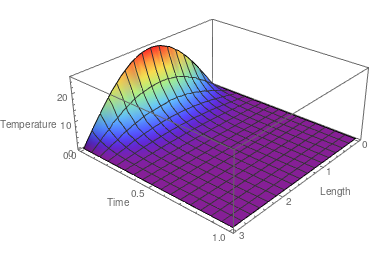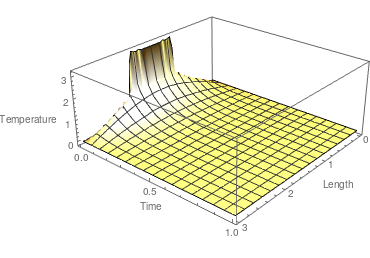Both figures, s0(x, t) at the left and u30(x, t) at the right, exhibit similar behavior for moderate t. The ripples near the edge t = 0 in the right-hand side figure indicate the Gibbs phenominon because of poor convergence of the series. To get rid of them, we apply >Cesàro summation.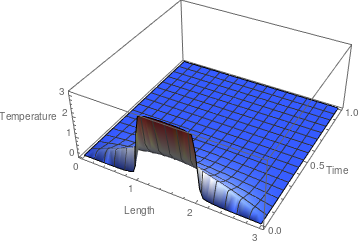We take the ;Cesàro truncated sum with 31 terms and plot it. As it is clearly seen, there is no Gibbs phenomenon. C30[x_, t_] = 2*Sum[(1 - k/31)*Exp[-(2*k + 1)^2*Pi^2*t]* Sin[(2*k + 1)*Pi* x/3]*(Cos[(2*k + 1)*Pi/3] - Cos[2*(2*k + 1)*Pi/3])/(2*k + 1), {k, 0, 30}]; Plot3D[C30[x, t], {x, 0, 3}, {t, 0, 1} , ColorFunction -> "TemperatureMap" , PlotRange -> All , AxesLabel -> {"Length", "Time", "Temperature"}] Cesàro summation for temperature approximation. Mathematica code

We plot temperature as a function of the position across the bar at different time values. In order to understand the influence of the first (main) term, we plot truncated versions of regular Fourier approximation and the corresponding first term for several values of t. Starting with t = 0.01, there is no visible difference in regular Fourier summation and corresponding Cesàro approximation.
Plot[{Callout[u30[x, 0.2], "u30(x,0.2)", LabelStyle -> {Bold, Orange}], Callout[s0[x, 0.2], "first term", LabelStyle -> {Bold, Blue}]}, {x, 0, 3}, PlotStyle -> Thickness[0.01]]
Plot[{Callout[u30[x, 1.2], "u30(x,1.2)", LabelStyle -> {Bold, Orange}], Callout[s0[x, 1.2], "first term", LabelStyle -> {Bold, Blue}]}, {x, 0, 3}, PlotStyle -> Thickness[0.01]]
Plot[{Callout[u30[x, 2.2], "u30(x,2.2)", LabelStyle -> {Bold, Orange}], Callout[s0[x, 2.2], "first term", LabelStyle -> {Bold, Blue}]}, {x, 0, 3}, PlotStyle -> Thickness[0.01]]
Of course, Mathematia complains that the exponential terms are too small for actual calculations. Therefore, 30-term approximation is not needed and first few terms is sufficient for accurate approximations starting at t = 1.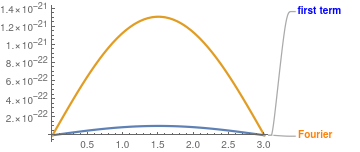Temperature distribution at t = 0.2. Temperature distribution at t = 1.2. Temperature distribution at t = 2.2.

In order plot the temperature as a function of the time at different position values along the bar, we compare its distribution with just first term.
$s_0 (x,t) = \frac{80}{\pi}\, e^{-\pi^2 t} \sin \left( \frac{\pi x}{3} \right) .$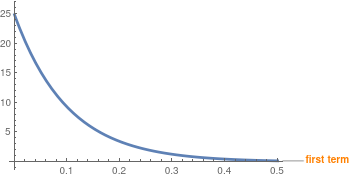We choose a typical point x = 1.7 and plot the truncated series with 30 terms along with its first term, s0(1.7, t). Plot[{Callout[s0[1.7, t], "first term", LabelStyle -> {Bold, Orange}], Callout[u0[1.7, t], "u30(1.7, t)", LabelStyle -> {Bold, Blue}]}, {t, 0, 0.5}, PlotStyle -> Thickness[0.01]] Temperature distribution with time at x = 1.7. Mathematica code

Finally, we demonstrate annimation for temperature distribution with time.
animation2 = Table[Plot[u30[x, t], {x, 0, 3}, PlotRange -> {0, 20}, PlotStyle -> Thickness[0.01] ], {t, 0, 0.5, 0.01}];
Export["1BAnimation.gif", animation2, "AnimationRepetitions" -> $Infinity]] ■ Example 1C: Consider the initial boundary value problem (IBVP) when the initial condition satisfies the regularity conditions: \begin{equation*} \begin{split} &\text{(PDE)} \quad u_{t} = 9\, u_{xx} , \quad 0<x<3, \ 0< t\le t^{\ast} < \infty , \\ &\text{(BC)} \hspace{6.2mm} u(0,t) =0 , \quad u (3 ,t) =0 , \quad 0< t\le t^{\ast} < \infty , \\ &\text{(IC)} \qquad u(x,0^{+}) = x^2 \left( 3-x \right) . \end{split} \end{equation*} Its solution, according to Eq.\eqref{EqBheat.3}, is \[ u(x,t) = \sum_{n\ge 1} c_n e^{-n^2 \pi^2 t} \sin \frac{n\pi x}{3} ,$
where
$c_n = - \frac{2}{3} \int_0^3 x^2 \left( 3-x \right) \sin \frac{n\pi x}{3} \,{\text d} x = \frac{108}{n^3 \pi^3} \left[ 1 + 2\cos \left( n\pi \right) \right] , \qquad n=1,2,3,\ldots .$
2*Integrate[x^2 *(3 - x) Sin[n*Pi*x/3], {x, 0, 3}]/3
-((54 (2 n $Pi] + 4 n \[Pi] Cos[n \[Pi]] + (-6 + n^2 \[Pi]^2) Sin[n \[Pi]]))/( n^4 \[Pi]^4)) Since the initial temperature distribution u(x, 0+) is a continuous function and satisfies the regularity conditions at the boundary. Therefore, the corresponding series solution converges uniformely for t ≥ 0. f3[x_] = x^2*(3 - x); cn = 2/3*Integrate[f3[x]*Sin[n*Pi*x/3], {x, 0, 3}]; computedSoln = Sum[-54 (2 n \[Pi] + 4 n \[Pi] Cos[n \[Pi]] + (-6 + n^2 \[Pi]^2) Sin[ n \[Pi]])/(n^4 \[Pi]^4)*Exp[-n^2*Pi^2*t]*Sin[n*Pi*x/3], {n, 1, Infinity}] Using Mathematica, we solve the heat equation with the appropriate boundary conditions. heatEq = D[u[x, t], t] == 9 * D[u[x, t], {x, 2}] heatEqSoln = u[x, t] /. DSolve[{heatEq, u[0, t] == 0, u[3, t] == 0, u[x, 0] == f3[x]}, u[x, t], {x, t}][[1, 1]] /. K -> n Since its hard to tell whether those two series are identical, lets take the first few terms of each to compare. Sum[-54 (2 n \[Pi] + 4 n \[Pi] Cos[n \[Pi]] + (-6 + n^2 \[Pi]^2) Sin[ n \[Pi]])/(n^4 \[Pi]^4)*Exp[-n^2*Pi^2*t]*Sin[n*Pi*x/3], {n, 1, 4}] As we see, the series are the same.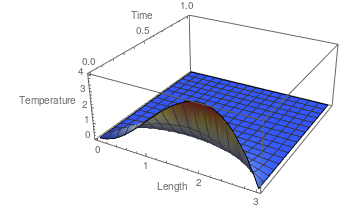We plot the solution approximation formed by adding the first 30 terms. First, we calculate the sum. heatEqSoln30C[x_, t_] = Sum[-54 (2 n \[Pi] + 4 n \[Pi] Cos[n \[Pi]] + (-6 + n^2 \[Pi]^2) Sin[ n \[Pi]])/(n^4 \[Pi]^4)*Exp[-n^2*Pi^2*t]*Sin[n*Pi*x/3], {n, 1, 30}]; Plot the solution for the length of the bar and the first second. Plot3D[heatEqSoln30C[x, t], {x, 0, 3}, {t, 0, 1} , ColorFunction -> "TemperatureMap" , PlotRange -> All , AxesLabel -> {"Length", "Time", "Temperature"}] 30-term temperature approximation. Mathematica code Plot temperature as a function of the position across the bar at different time values. Plot[heatEqSoln30C[x, 0.01], {x, 0, 3}, PlotStyle -> Thickness[0.01]] Plot[heatEqSoln30C[x, 0.1], {x, 0, 3}, PlotStyle -> Thickness[0.01]] Plot[heatEqSoln30C[x, 0.5], {x, 0, 3}, PlotStyle -> Thickness[0.01]]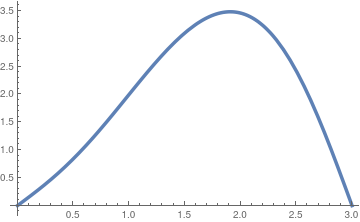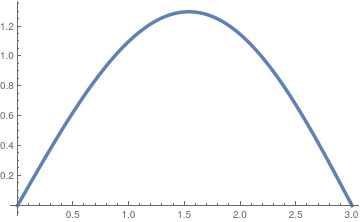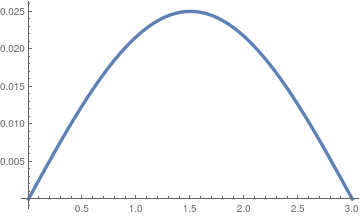Temperature distribution at t = 0.01. Temperature distribution at t = 0.1. Temperature distribution at t = 0.5. Produce an animation of the temperature in the rod as time progresses. animation3 = Table[Plot[heatEqSoln30C[x, t], {x, 0, 3}, PlotRange -> {0, 4}, PlotStyle -> Thickness[0.01] ], {t, 0, 0.1, 0.001}]; Export["1CAnimation.gif", animation3, "AnimationRepetitions" -> \[Infinity]] ■ Example 1D: Consider the initial boundary value problem with specific initial temperature distribution: \begin{equation*} \begin{split} &\text{(PDE)} \quad u_{t} = 9\, u_{xx} , \quad 0<x<3, \ 0< t\le t^{\ast} < \infty , \\ &\text{(BC)} \hspace{6.2mm} u(0,t) =0 , \quad u (3 ,t) =0 , \quad 0< t\le t^{\ast} < \infty , \\ &\text{(IC)} \qquad u(x,0^{+}) = \sin (\pi x) - 3\,\sin (2\pi x) . \end{split} \end{equation*} In the given problem, we consider a bar of length ℓ = 3 with thermal diffusivity α = 9. We can find its solution using the general formula \eqref{EqBheat.3} and evaluate coefficients according to \eqref{EqBheat.5}. However, we don't need to go over this tedious procedure because the initial condition function u(x, 0+) = f(x) is a linear combination of the eigenfunctions. Since the sine Fourier series expansion is unique for any square integrable function f ∈ 𝔏², this function f(x) is its Fourier series for itself: \[ \sin (\pi x) - 3\,\sin (2\pi x) = 0 \cdot \sin \frac{\pi x}{3} + 0 \cdot \sin \frac{2\pi x}{3} + 1 \cdot \sin \frac{3\pi x}{3} + 0 \cdot \sin \frac{4\pi x}{3} + 0 \cdot \sin \frac{5\pi x}{3} - 2 \cdot \sin \frac{6\pi x}{3} + 0 \cdot \sin \frac{7\pi x}{3} + \cdots .$
This allows us to identify the coefficients to be all zeroes except two indices: c3 = 1 and c6 = −3. Using the superposition principle, the solution of the given IBVP can be obtained by adding together two simpler solutions corresponding n = 3 and n = 6 obtained by the product method:
$u(x,t) = e^{-9\pi^2 t} \,\sin (\pi x) - 3\,e^{-36 \pi^2 t} \,\sin (2\pi x) .$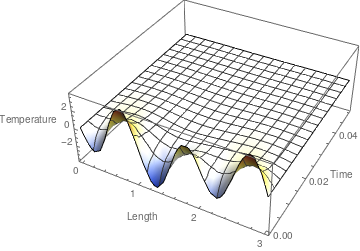We plot the solution. heat1D[x_, t_] = Exp[-9*Pi^2*t]*Sin[Pi*x] - 3*Exp[-36*Pi^2*t]*Sin[2*Pi*x]; Plot3D[heat1D[x, t], {x, 0, 3}, {t, 0, .05} , ColorFunction -> "TemperatureMap" , PlotRange -> All , AxesLabel -> {"Length", "Time", "Temperature"}] Plot the solution for the length of the bar and the first second. Plot3D[heatEqSoln30C[x, t], {x, 0, 3}, {t, 0, 1} , ColorFunction -> "TemperatureMap" , PlotRange -> All , AxesLabel -> {"Length", "Time", "Temperature"}] Temperature distribution. Mathematica code

Produce an animation of the temperature in the rod as time progresses
nimation4 = Table[Plot[heat1D[x, t], {x, 0, 3}, PlotRange -> {-4, 4}, PlotStyle -> Thickness[0.01] ], {t, 0, 0.05, 0.001}];
Export["1DAnimation.gif", animation4, "AnimationRepetitions" -> $Infinity]] ■ Rod with both ends insulated (Neumann conditions) Let us consider the situation where two ends of the bar are also sealed with perfect insulation so that no heat can escape to the outside environment (recall that the side of the bar is always perfectly insulated in the one-dimensional assumption), or vice versa. The new boundary conditions follow from the Fourier’s law of heat transfer that the rate of heat transfer is proportional to negative temperature gradient: \[ \frac{\mbox{Rate}\ \mbox{ of }\ \mbox{ heat}\ \mbox{ transfer}}{\mbox{area}} = -\kappa\,\nabla u = - \kappa\,\frac{\partial u}{\partial x} ,$
where κ is the thermal conductivity. In other words, heat is transferred from areas of high temperature to low temperature.
$\left. \frac{\partial u}{\partial x} \right\vert_{x=0} = 0 \qquad \mbox{and} \qquad \left. \frac{\partial u}{\partial x} \right\vert_{x=\ell} = 0,$ reflecting the fact that there will be no heat transferring, spatially, across the points x = 0 and x = ℓ. The heat conduction problem becomes the initial-boundary value problem below.
\begin{align*} &\mbox{Heat equation:} \qquad &u_t &= \alpha\,u_{xx} \qquad\mbox{for } 0 < x < \ell \mbox{ and } t > 0, \\ &\mbox{Boundary condition:} \qquad &u_x (0,t) &= 0 , \quad u_x (\ell , t) = 0, \\ &\mbox{Initial condition:} \qquad &u(x,0^{+}) &= f(x) , \qquad 0 < x < \ell , \qquad t > 0 . \end{align*}
The first step is to temporarily disregard the initial condition (because it is non-homogeneous) and consider an auxiliary boundary value problems. Next, we apply the separation of variables for the function u(x, t) = X(x) T(t )that leads to two ordinary differential equations
\begin{align*} T'(t) + \alpha \lambda\,T(t) &= 0, \\ X'' (x) + \lambda\,X(x) &= 0 , \end{align*}
where primes stand for derivatives (Lagrange's notation). The homogeneous Neumann boundary conditions are also separated into
$\begin{split} u_x (0,t) = X'(0)\,T(t) = 0 \qquad \Longrightarrow \qquad X'(0) = 0 , \\ u_x (\ell ,t) = X'(\ell )\,T(t) = 0 \qquad \Longrightarrow \qquad X'(\ell ) = 0 , \end{split}$
This leads to the eigenvalue problem
$X'' (x) + \lambda\,X(x) = 0, \qquad X' (0) = 0, \quad X' (\ell ) = 0.$
Let us first show that λ = 0 is an eigenvalue. Indeed, the general solution of the differential equation with this λ is
$X(x) = c_1 + c_2 x \qquad \Longrightarrow \qquad X' (x) = c_2 .$
To satisfy the Neuman boundary conditions, we have to set c2 = 0. Then function $$X(x) = c_1$$ is a solution of the differential equation $$X'' (x) + 0\cdots X(x) = 0$$ and automatically satisfies both Neuman boundary conditions. Therefore, any constant solution X(x) = c1 ≠ 0 is an eigenfunction.

Assuming λ to be positive, the general solution of the differential equation for X(x) becomes

$X(x) = c_1 \cos \left( \sqrt{\lambda}\,x \right) + c_2 \sin \left( \sqrt{\lambda}\,x \right) ,$
with some constants c1 and c2. Taking derivative $$X'(x ) = \sqrt{\lambda} \left[ -c_1 \sin \left( \sqrt{\lambda}\,x \right) + c_2 \cos \left( \sqrt{\lambda}\,x \right) \right] ,$$ and substituting into the boundary conditions, we get
$c_2 =0 , \qquad \sqrt{\lambda} \,c_1 \sin \left( \sqrt{\lambda}\,\ell \right) =0.$
Since λ is positive (by assumption), the only choice we have is to set $$\sin \left( \sqrt{\lambda}\,\ell \right) =0$$ because we cannot affort a trivial solution by setting c2 = 0. Then we obtain the eigenvalues and corresponding eigenfunctions:
$\lambda_n = \left( \frac{n\pi}{\ell} \right)^2 , \quad X_n = \cos \left( \frac{n\pi x}{\ell} \right) , \qquad n=0,1,2,\ldots .$
We included n = 0 because λ = 0 is an eigenvalue with a constant as an eigenfunction. Every auxiliary function un(x, t) = Xn(x) is a solution of the homogeneous heat equation \eqref{EqBheat.1} and satisfy the homogeneous Neumann boundary conditions. Thereofre, any their linear combination will also a solution of the heat equation subject to the Neumann boundary conditions. Hence we expect the sum over all auxiliary partial nontrivial solutions will be a solution of our given IBVP provided that the infinite series converges. So we seek the solution in the form:
\begin{equation} \label{EqBheat.6} u(x,t) = \frac{a_0}{2} + \sum_{n\ge 1} e^{- \alpha \lambda_n t} \cos \left( \frac{n\pi x}{\ell} \right) , \end{equation}
where coefficients cn are obtained from expansion of f(x) into Fourier cosine series:
\begin{equation} \label{EqBheat.7} c_n = \frac{2}{\ell} \int_0^{\ell} f(x)\,\cos \left( \frac{n\pi x}{\ell} \right) {\text d} x , \qquad n=0,1,2,\ldots . \end{equation}

Example 2A: Consider the initial boundary value problem (IBVP):

\begin{align*} &\mbox{Heat equation:} \qquad &u_t &= \frac{1}{4}\,u_{xx} \qquad\mbox{for } 0 < x < 10 \quad \mbox{and} \quad t > 0, \\ &\mbox{Boundary condition:} \qquad &u_x (0,t) &= 0 , \quad u_x (10 , t) = 0, \qquad t > 0 , \\ &\mbox{Initial condition:} \qquad &u(x,0^{+}) &= x^2 \left( 15 -x \right) , \qquad 0 < x < 10 . \end{align*}
The initial temperature f(x) = x²(15 −x) satisfies the regularity conditions: $$f' (0) = f' (10) = 0 .$$ Therefore, we expect that the given IBVP to be well-posed. Since its solution is available from Eq.\eqref{EqBheat.6}, we get the explicit fornmula for it:
$u(x,t) = \frac{a_0}{2} + \sum_{n\ge 1} c_n e^{- \alpha \lambda_n t} \cos \left( \frac{n\pi x}{10} \right) , \qquad \lambda_n = \left( \frac{n\pi}{10} \right)^2 , \qquad \alpha = \frac{1}{4} ,$
where
$c_0 = \frac{2}{10} \int_0^{10} x^2 \left( 15 -x \right) {\text d} x = 500$
and
$c_n = \frac{1}{5} \int_0^{10} x^2 \left( 15 -x \right) \cos \frac{n\pi x}{10}\,{\text d} x =$
$c_n = \frac{1}{5} \int_0^{10} x^2 \left( 15 -x \right) \cos \left( \frac{n\pi x}{10}\right) {\text d} x = -\frac{12000}{n^4 \pi^4} \left( -1 + (-1)^n \right) = \frac{24000}{\pi^4} \times \begin{cases} \frac{1}{(2k+1)^4} , & \ \mbox{ for } n =2k+1 , \\ 0, & \ \mbox{otherwise} . \end{cases}$
Integrate[x^2*(15 - x), {x, 0, 10}]/5
500
Assuming[Element[n, Integers], Integrate[x^2 *(15 - x)*Cos[n*Pi*x/10], {x, 0, 10}]/5]
(12000 (-1 + (-1)^n))/(n^4 $Pi]^4) Therefore, \[ u(x,t) = 250 - \frac{24000}{\pi^4} \sum_{k\ge 0} \frac{1}{(2k+1)^4}\, e^{-(2k+1)^2 \pi^2 t/400} \cos \frac{\left( 2k+1 \right) \pi x}{10} .$
Since the series solution converges rapidly, we can take only 15 terns for accurate approximation of the solution.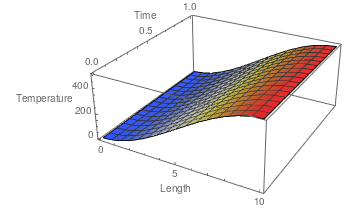We plot the temperature distribution inside the bar. u15[x_, t_] = 250 - 24000/Pi^4 * Sum[Exp[-(2*k + 1)^2 *Pi^2 *t/400]* Cos[(2*k + 1)*Pi*x/10]/(2*k + 1)^4, {k, 0, 15}]; Plot3D[u15[x, t], {x, 0, 10}, {t, 0, 1}, ColorFunction -> "TemperatureMap", PlotRange -> All, AxesLabel -> {"Length", "Time", "Temperature"}] Temperature distribution. Mathematica code

■

Example 2B: Consider the initial boundary value problem (IBVP):

\begin{align*} &\mbox{Heat equation:} \qquad &u_t &= \frac{1}{4}\,u_{xx} \qquad\mbox{for } 0 < x < 10 \quad \mbox{and} \quad t > 0, \\ &\mbox{Boundary condition:} \qquad &u_x (0,t) &= 0 , \quad u_x (10 , t) = 0, \qquad t > 0 , \\ &\mbox{Initial condition:} \qquad &u(x,0) &= H(x-5) - H(x-8) , \end{align*}
where H(x) is the Heaviside function. Although the initial temperature function satisfies the regularity conditions, it is piecewise continous. Therefore, we expect to observe a Gibbs phenomenon dues to poor convergence of the corresponding solution series.

According the previous example, its solution is

$u(x,t) = \frac{a_0}{2} + \sum_{n\ge 1} c_n e^{- \alpha \lambda_n t} \cos \left( \frac{n\pi x}{10} \right) , \qquad \lambda_n = \left( \frac{n\pi}{10} \right)^2 , \qquad \alpha = \frac{1}{4} ,$
where
$c_0 = \frac{2}{10} \int_5^{8} {\text d} x = \frac{3}{5}$
and
$c_n = \frac{1}{5} \int_5^{8} \cos \frac{n\pi x}{10}\,{\text d} x = \frac{2}{n \pi} \left( \sin \frac{n\pi}{2} - \sin \frac{4n\pi}{5} \right) , \qquad n=1,2,\ldots .$
Integrate[1, {x, 5, 8}]/5
3/5
Integrate[Cos[n*Pi*x/10], {x, 5, 8}]/5
(2 (-Sin[(n $Pi])/2] + Sin[(4 n \[Pi])/5]))/(n \[Pi]) So \[ u(x,t) = \frac{3}{10} - \frac{2}{\pi} \sum_{n \ge 1} \frac{1}{n}\, e^{-n^2 \pi^2 t/400} \cos \frac{n\pi x}{10} \left( \sin \frac{n\pi}{2} - \sin \frac{4n\pi}{5} \right) .$
The series converges uniformly in any domain tt0 > 0 because of the expobnentially decayed multiple containing t. However, when t is close to zero, the series converges conditionally and the initial condition is not easy to satisfy. To suppress the Gibbs phenomenon, we apply the Cesàro summation. First, we define a 31-term approximation
u30[x_,t_] = 3/10 - 2*Sum[Exp[-n^2 * Pi^2 *t/400]*Cos[n*Pi*x/10] * (Sin[n*Pi/2] - Sin[4*n*Pi/5])/n, {n,1,30}]/5;
and its Cesàro counterpart
C30[x_,t_] = 3/10 - 2*Sum[(1-n/31)*Exp[-n^2 * Pi^2 *t/400]*Cos[n*Pi*x/10] * (Sin[n*Pi/2] - Sin[4*n*Pi/5])/n, {n,1,30}]/5;
Then we plot both functions.
Plot3D[u30[x, t], {x, 0, 10}, {t, 0, 1}, ColorFunction -> "TemperatureMap", PlotRange -> All, AxesLabel -> {"Length", "Time", "Temperature"}]
and
Plot3D[C30[x, t], {x, 0, 10}, {t, 0, 1}, ColorFunction -> "Rainbow", PlotRange -> All, AxesLabel -> {"Length", "Time", "Temperature"}]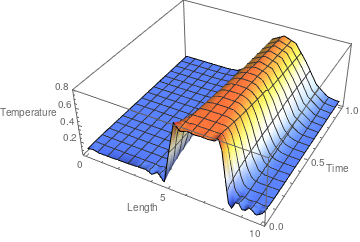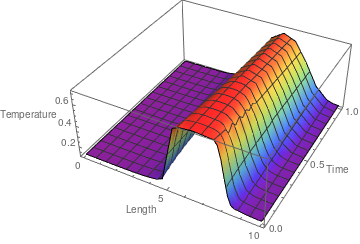Temperature distribution. Cesàro approximation.

The Gibbs phenomenon is clearly observed from the right-hand side figure.     ■

Example 2C: Consider the initial boundary value problem with specific initial temperature distribution:

\begin{equation*} \begin{split} &\text{(PDE)} \quad u_{t} = \frac{1}{4}\, u_{xx} , \quad 0<x<10, \ 0< t\le t^{\ast} < \infty , \\ &\text{(BC)} \hspace{6.2mm} u_x (0,t) =0 , \quad u_x (10 ,t) =0 , \quad 0< t\le t^{\ast} < \infty , \\ &\text{(IC)} \qquad u(x,0^{+}) = \cos (\pi x) - 3\,\cos (2\pi x) . \end{split} \end{equation*}
In the given problem, we consider a bar of length ℓ = 10 with thermal diffusivity α = ¼. We can find its solution using the general formula \eqref{EqBheat.6} and evaluate coefficients according to \eqref{EqBheat.7}. However, we don't need to go over this tedious procedure because the initial condition function u(x, 0+) = f(x) is a linear combination of the eigenfunctions. Since the cosine Fourier series expansion is unique for any square integrable function f ∈ 𝔏², this function f(x) = cos(πx) −2 cos(2πx)is its Fourier series for itself:
$\cos (\pi x) - 3\,\cos (2\pi x) = 0 \cdot 1 + 0 \cdot \cos \frac{\pi x}{10} + 0 \cdot \cos \frac{2\pi x}{10} + \cdots + 1 \cdot \cos \frac{10\pi x}{10} + 0 \cdot \cos \frac{4\pi x}{10} + \cdots + 0 \cdot \cos \frac{19\pi x}{10} - 2 \cdot \cos \frac{20\pi x}{10} + 0 \cdot \cos \frac{21\pi x}{10} + \cdots .$
This allows us to identify the coefficients to be all zeroes except two indices: c10 = 1 and c20 = −3. Using the superposition principle, the solution of the given IBVP can be obtained by adding together two simpler solutions corresponding n = 3 and n = 6 obtained by the product method:
$u(x,t) = e^{-25\pi^2 t} \,\cos (\pi x) - 3\,e^{-100 \pi^2 t} \,\cos (2\pi x) .$
■

Simple Mixed boundary condition for the diffusion equation

We consider two initial boundary value problems with Dirichlet's condition (when temperature is prescribed to be zero) on one end and Neumann condition (which is equivalent insulation) on another end.

Problem DN:

\begin{align*} &\mbox{Heat equation:} \qquad &u_t &= \alpha\,u_{xx} \qquad\mbox{for } 0 < x < \ell \mbox{ and } t > 0, \\ &\mbox{Boundary condition:} \qquad &u (0,t) &= 0 , \quad u_x (\ell , t) = 0, \qquad t > 0 , \\ &\mbox{Initial condition:} \qquad &u(x,0^{+}) &= f(x) , \qquad 0 < x < \ell , \\ &\mbox{Regularity conditions:} \qquad &f(0) &= u (0,0) = 0 , \quad f'(\ell ) = u_x (\ell , 0) = 0, \qquad \mbox{and} \qquad \lim_{t\to +\infty} u(x,t) = 0. \end{align*}
When regularity conditions are satisfied, the given initial boundary value problem (IBVP for short) is well-posed. However, when the conditions at end points do not meet or the initial temperature is modelled by a ppiecewise continuous function, the IBVP can also be solved, but it becomes ill-posed and numerical calculations must be done with care.

Disregarding the initial condition, we obtain an auxiliary boundary value problem. We seek for its non-trivial solutions that are represented as the product u(x, t) = X(x) T(t) of two functions, X(x) and T(t), each of which depends on single independent variable. Substituting this form into the heat equation, we get

$T' (t)\, X(x) = \alpha\, T(t)\,X'' (x) \qquad \Longrightarrow \qquad \frac{\dot{T} (t)}{\alpha\,T(t)} = \frac{X'' (x)}{X(x)} .$
Its left-hand side depends on time variable t, but the right-hand side depends on spatial variable x that are independent. Therefore, this equation holds only when both sides are constants. As usual, we denot this constant as −λ. This give two differential equations containing a parameter λ (which is unknown yet):
$\dot{T} (t) + \alpha\,\lambda \,T(t) = 0 \qquad \mbox{and} \qquad X'' (x) + \lambda \,X(x) = 0 .$
From the homogeneous boundary conditions, we get
$u(0,t) = X(0)\,T(t) = 0 \qquad \Longrightarrow \qquad X(0) = 0 ,$
and
$u_x (\ell ,t) = X'(\ell )\,T(t) = 0 \qquad \Longrightarrow \qquad X'(\ell ) = 0 .$
The boundary conditions for X(x) together with the second order differential equation form so called the Sturm--Liuville problem:
$X'' (x) + \lambda \,X(x) = 0 , \qquad X(0) = 0 , \quad X' (\ell ) = 0.$
It can be shown that the Sturm--Liouville problem has nontrivial solutions only for positive values of parameter λ. Assuming this, we obtain the general solution to the differential equation $$X'' (x) + \lambda\,X(x) =0$$ to become
$X(x) = c_1 \cos \left( \sqrt{\lambda}\,x \right) + c_2 \sin \left( \sqrt{\lambda}\,x \right) .$
To satisfy the boundary condition at x = 0 we have to set c₁ = 0 and obtain $$X(x) = c_2 \sin \left( \sqrt{\lambda}\,x \right) .$$ An arbitrary constant c₂ ≠ 0 in order to avoid the identically zero solution, so it can be dropped. Next substitution of this function into the boundary condition at x = ℓ, we get
$X' (\ell ) = c_2 \sqrt{\lambda} \,\cos \left( \sqrt{\lambda}\,\ell \right) = 0 \qquad \Longrightarrow \qquad \cos \left( \sqrt{\lambda}\,\ell \right) = 0$
because λ > 0 (by assumption) and c₂ ≠ 0 to avoid trivial solution. Therefore, λ must be a root of the transcendent equation
$\cos \left( \sqrt{\lambda}\,\ell \right) = 0 \qquad \Longrightarrow \qquad \lambda = \lambda_n = \left( \frac{\pi \left( 2n+1 \right)}{2\ell} \right)^2 , \qquad n=0,1,2,\ldots .$
With these eigenvalues, we obtain eigenfunctions to be
$X_n (x) = \sin \left( \frac{\pi \left( 2n+1 \right) x}{2\ell} \right) , \qquad n=0,1,2,\ldots .$
Substituting λ = λN into the differential equation for T(t), $$T' (t) + \alpha\lambda\, T(t) = 0 ,$$ we get
$T(t) = T_n (t) = c_n e^{-\alpha \lambda_n t} ,$
with some arbitrary constant cn. Multiplying Tn(t) and Xn(x), we find partial nontrivial solutions to our auxiliary boundary value problem. Upon summing all these partial nontrivial solutions, we represent the required solution as an infinite series
\begin{equation} \label{EqBheat.8} u(x,t) = \sum_{n\ge 0} c_n e^{-\alpha \lambda_n t} \sin \left( \frac{\pi \left( 2n+1 \right) x}{2\ell} \right) , \qquad \lambda_n = \left( \frac{\pi \left( 2n+1 \right)}{2\ell} \right)^2 . \end{equation}
Every term in this infinite series satisfies the heat conduction equation and both boundary conditions. Assuming that this series converges, we extend this statement for the series. Now substituting u(x, t) into the initial condition,
$\lim_{t\downarrow 0} u(x,t) = u(x,0^{+}) = \sum_{n\ge 0} \lim_{t\downarrow 0} c_n e^{-\alpha \lambda_n t} \sin \left( \frac{\pi \left( 2n+1 \right) x}{2\ell} \right) = \sum_{n\ge 0} c_n \sin \left( \frac{\pi \left( 2n+1 \right) x}{2\ell} \right) .$
Equating the right-hand side to f(f), we obtain
$\lim_{t\downarrow 0} u(x,t) = f(x) = \sum_{n\ge 0} c_n \sin \left( \frac{\pi \left( 2n+1 \right) x}{2\ell} \right) .$
This identity is valid only when the limit can be evaluated under the sum sign (which is true when the series converges uniformly). It is not our case, and we apply the Cesàro summation to prove the validity of interchanging the order of limiting procedure and summation.
$u(x,t) = \lim_{N \to \infty} \, \sum_{n=0}^{N} \left( 1 - \frac{n}{N+1} \right) c_n e^{-\alpha \lambda_n t} \sin \left( \frac{\pi \left( 2n+1 \right) x}{2\ell} \right) .$
The coefficients in this expansion are known to be
\begin{equation} \label{EqBheat.9} c_n = \frac{2}{\ell} \int_0^{\ell} f(x) \, \sin \left( \frac{\pi \left( 2n+1 \right) x}{2\ell} \right) {\text d} x , \qquad n=0,1,2,\ldots . \end{equation}

Example 3A: Consider the initial boundary value problem (IBVP) for a rod of length 3 with thermal diffusivity 9, and mixed boundary conditions:

\begin{align*} &\mbox{Heat equation:} \qquad &u_t &= 9\,u_{xx} \qquad\mbox{for } 0 < x < 3 \mbox{ and } t > 0, \\ &\mbox{Boundary condition:} \qquad &u (0,t) &= 0 , \quad u_x (3 , t) = 0, \qquad t > 0 , \\ &\mbox{Initial condition:} \qquad &u(x,0^{+}) &= x\left( 6-x \right) , \qquad 0 < x < 3 . \end{align*}
Note the the regularity conditions are valid, so the given problem is well-posed. We seek its solution as the sum of all partial nontrivial solutions (assuming that the series converges uniformely):
$u(x,t) = \sum_{n\ge 0} c_n e^{-9 \lambda_n t} \sin \left( \frac{\pi \left( 2n+1 \right) x}{6} \right) , \qquad \lambda_n = \left( \frac{\pi \left( 2n+1 \right)}{6} \right)^2 .$
In order to satisfy the initial conditions, we take the limit as t approaches zero; assuming that the series converges uniformly. Then we apply the limit to every term under the summation sign and obtain
$\lim_{t \downarrow 0} u(x,t) = u(x,t^{+}) = \sum_{n\ge 0} c_n \sin \left( \frac{\pi \left( 2n+1 \right) x}{6} \right) = f(x) = x\left( 6-x \right) ,$
which is the Fourier series. So the coefficients are known to be
$c_n = \frac{2}{3} \int_0^3 x\left( 6-x \right) \sin \left( \frac{\pi \left( 2n+1 \right) x}{6} \right) {\text d} x , \qquad n=0,1,2,\ldots .$
Using Mathematica, we get the numerical values
cn = Assuming[Element[n, Integers], 2*Integrate[x*(6 - x)*Sin[Pi*x*(2*n + 1)/6], {x, 0, 3}]/3]
288/($Pi] + 2 n \[Pi])^3 Therefore, the solution of the given IBVP becomes \[ u(x,t) = \frac{288}{\pi^3} \,\sum_{n\ge 0} \frac{1}{(2n+1)^3} \, e^{-(2n+1)^2 \pi^2 t/4} \sin \left( \frac{\pi \left( 2n+1 \right) x}{6} \right) .$
Taking a truncated sum with 20 terms as a proper approximation, we use Mathematica for plotting.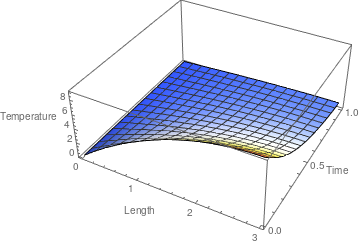We plot the temperature distribution inside the bar. dn20[x_, t_] = 288/(Pi)^3 * Sum[Exp[-(2*n + 1)^2 *Pi^2 *t/4]* Sin[(2*n + 1) *Pi*x/6]/(2*n + 1)^3, {n, 0, 20}]; Plot3D[dn20[x, t], {x, 0, 3}, {t, 0, 1} , ColorFunction -> "TemperatureMap" , PlotRange -> All , AxesLabel -> {"Length", "Time", "Temperature"}] Temperature distribution. Mathematica code

Now we plot temperature as a function of the position across the bar at different time values.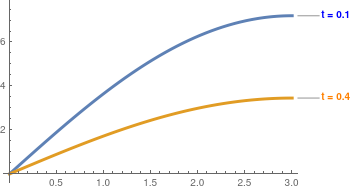We plot the temperature distribution inside the bar at t = 0.1 and t = 0.4. Plot[{Callout[dn20[x, 0.1], "t = 0.1", LabelStyle -> {Bold, Blue}], Callout[dn20[x, 0.4], "t = 0.4", LabelStyle -> {Bold, Orange}]}, {x, 0, 3}, PlotStyle -> Thickness[0.01]] Temperature inside the bar at t = 0.1 and 0.4. Mathematica code

Next, we repeat calculations for x = 1 and x = 2.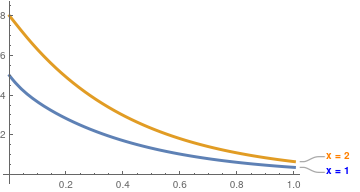We plot the temperature distribution inside the bar at x = 1 and x = 2. Plot[{Callout[dn20[1, t], "x = 1", LabelStyle -> {Bold, Blue}], Callout[dn20[2, t], "x = 2", LabelStyle -> {Bold, Orange}]}, {t, 0, 1}, PlotStyle -> Thickness[0.01]] Temperature inside the bar at t = 0.1 and 0.4. Mathematica code

■

Example 3B: We consider a similar problem with a constant initial temperature when the regularity conditions are not met.

\begin{align*} &\mbox{Heat equation:} \qquad &u_t &= 9\,u_{xx} \qquad\mbox{for } 0 < x < 3 \mbox{ and } t > 0, \\ &\mbox{Boundary condition:} \qquad &u (0,t) &= 0 , \quad u_x (3 , t) = 0, \qquad t > 0 , \\ &\mbox{Initial condition:} \qquad &u(x,0^{+}) &= 21 , \qquad 0 < x < 3 . \end{align*}
Its solution, according to Eq.\eqref{EqBheat.8}, is
$u(x,t) = \sum_{n\ge 0} c_n e^{-9 \lambda_n t} \sin \left( \frac{\pi \left( 2n+1 \right) x}{6} \right) , \qquad \lambda_n = \left( \frac{\pi \left( 2n+1 \right)}{6} \right)^2 ,$
where
$c_n = \frac{2}{3} \int_0^3 21\, \sin \left( \frac{\pi \left( 2n+1 \right) x}{6} \right) {\text d} x = \frac{84}{\pi \left( 2n+1 \right)} , \qquad n=0,1,2,\ldots .$
Assuming[Element[n, Integers], 2*21/3*Integrate[Sin[(2*n + 1)*Pi*x/6], {x, 0, 3}]]
84/(Pi] + 2 n \[Pi]) Taking a truncated sum with 31 terms as a proper approximation, we use Mathematica for plotting.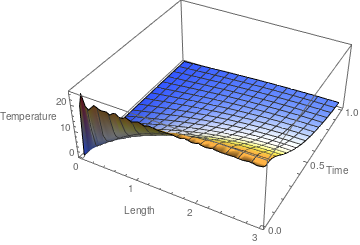We plot the temperature distribution inside the bar. u30[x_, t_] = 84*Sum[Exp[-(2*n+1)^2 *Pi^2 *t/4]*Sin[(2*n+1)*Pi*x/6]/(2*n+1), {n,0,30}]/Pi; Plot3D[u30[x, t], {x, 0, 3}, {t, 0, 1} , ColorFunction -> "TemperatureMap" , PlotRange -> All , AxesLabel -> {"Length", "Time", "Temperature"}] Temperature distribution. Mathematica code We see the Gibbs phenomenon near t = 0 because of poor convergence. We suppress ripples upon application of the Cesàro summation.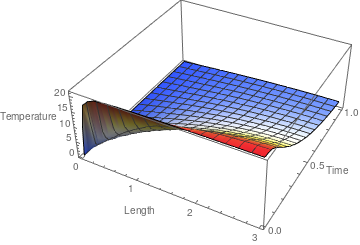We plot the temperature distribution inside the bar using Cesàro summation. C30[x_, t_] = 84*Sum[(1 - n/31)*Exp[-(2*n+1)^2 *Pi^2 *t/4]*Sin[(2*n+1)*Pi*x/6]/(2*n+1), {n,0,30}]/Pi; Plot3D[C30[x, t], {x, 0, 3}, {t, 0, 1} , ColorFunction -> "TemperatureMap" , PlotRange -> All , AxesLabel -> {"Length", "Time", "Temperature"}] Temperature distribution. Mathematica code ■ Problem ND: We consider a heat transfer problem for a rod of length ℓ with Dirichlet's condition (when temperature is prescribed to be zero) on right end x = ℓ and Neumann's condition (which is equivalent insulation) on another end x = 0. \begin{align*} &\mbox{Heat equation:} \qquad &u_t &= \alpha\,u_{xx} \qquad\mbox{for } 0 < x < \ell \mbox{ and } t > 0, \\ &\mbox{Boundary condition:} \qquad &u_x (0,t) &= 0 , \quad u (\ell , t) = 0, \qquad t > 0 , \\ &\mbox{Initial condition:} \qquad &u(x,0^{+}) &= f(x) , \qquad 0 < x < \ell , \\ &\mbox{Regularity conditions:} \qquad &f'(0) &= u_x (0,0) = 0 , \quad f(\ell ) = u (\ell , 0) = 0, \qquad \mbox{and} \qquad \lim_{t\to +\infty} u(x,t) = 0. \end{align*} Application of separation of variables leads to the Sturm--Liouville problem \[ X'' (x) + \lambda\,X(x) = 0, \qquad X' (0) = 0, \quad X(\ell ) = 0.
It has eigenfunctions
$X_n (x) = \cos \left( \frac{\left( 2n +1 \right) \pi x}{2\ell} \right) , \qquad\mbox{with} \quad \lambda_n = \left( \frac{\left( 2n +1 \right) \pi }{2\ell} \right)^2 .$
So the heat equation subject to the homogeneous boundary conditions has a sequence of nontrivial solutions:
$u_n (x,t) = X_n (x)\, T_n (t) = c_n e^{-\alpha \,\lambda_n t} \cos \left( \frac{\left( 2n +1 \right) \pi x}{2\ell} \right) , \qquad n=0,1,2,3,\ldots .$
Now solution of the given IBVP is the sum of all partial nontrivial solutions
\begin{equation} \label{EqBheat.10} u(x,t) = \sum_{n\ge 0} c_n e^{-\alpha \lambda_n t} \cos \left( \frac{\pi \left( 2n+1 \right) x}{2\ell} \right) , \qquad \lambda_n = \left( \frac{\pi \left( 2n+1 \right)}{2\ell} \right)^2 , \end{equation}
where the coefficients are
\begin{equation} \label{EqBheat.11} c_n = \frac{2}{\ell} \int_0^{\ell} f(x)\,\cos \left( \frac{\left( 2n +1 \right) \pi x}{2\ell} \right) {\text d}x , \qquad n=0,1,2,3,\ldots . \end{equation}

Example 3C:

\begin{align*} &\mbox{Heat equation:} \qquad &u_t &= \alpha\,u_{xx} \qquad\mbox{for } 0 < x < \ell \mbox{ and } t > 0, \\ &\mbox{Boundary condition:} \qquad &u_x (0,t) &= 0 , \quad u (\ell , t) = 0, \qquad t > 0 , \\ &\mbox{Initial condition:} \qquad &u(x,0) &= f(x) , \qquad 0 < x < \ell . \end{align*}
■

Example 3D: We consider the folloing IBVP:

\begin{align*} &\mbox{Heat equation:} \qquad &u_t &= 9\,u_{xx} \qquad\mbox{for } 0 < x < 3 \mbox{ and } t > 0, \\ &\mbox{Boundary condition:} \qquad &u_x (0,t) &= 0 , \quad u (3 , t) = 0, \qquad t > 0 , \\ &\mbox{Initial condition:} \qquad &u(x,0^{+}) &= H(x-1) - H(x-2) , \qquad 0 < x < 3 . \end{align*}
Here, H(x) is the Heaviside function.     ■

Boundary condition with convection

\begin{align*} &\mbox{Heat equation:} \qquad &u_t &= \alpha\,u_{xx} \qquad\mbox{for } 0 < x < \ell \mbox{ and } t > 0, \\ &\mbox{Boundary condition:} \qquad &u (0,t) &= 0 , \quad -\kappa\,u_x (\ell , t) = hA \left( u(\ell , t) - T_{\infty} \right) , \qquad t > 0 , \\ &\mbox{Initial condition:} \qquad &u(x,0) &= f(x) , \qquad 0 > x > \ell . \end{align*}
So the left end x = 0 is maintained with a constant (zero) temperature, while on the another end x = ℓ there is a convection heat transfer with surrounded air of temperature T. Since we are in the section to illustrate separation of variables method, we make a simplified assumption that T is zero. This leads to the following (simplified boundary conditions:
$u(0,t) = 0 , \qquad \left( u + \gamma\,\frac{\partial u}{\partial x} \right)_{x=\ell} = 0 .$
where γ is a positive constant. In order to apply the separation of variables method, we temporarily disregard the initial condition (because it it is nonhomogeneous) and obtain the auxiliary boundary value problem
$u_t = \alpha\,u_{xx} , \qquad u(0) = 0 , \quad \left( u + \gamma\,u_x \right)_{x=\ell} = 0 .$
Assuming that this problem has a solution represented as a product of two functions, u(x, t) = X(x) T(t), we obtain upon its substitution into the heat equation that
$X(x)\,T' (t) = \alpha\,X''(x)\, T(t) \qquad \Longrightarrow \qquad \frac{T' (t)}{\alpha \,T(t)} = \frac{X'' (x)}{X(x)} = -\lambda , \qquad\mbox{a constant}.$
Substituting the product u(x, t) = X(x) T(t) into the boundary conditions, we obtain
$X(0) \,T(t) = 0 \qquad \Longrightarrow \qquad X(0) = 0,$
and
$\left( X(\ell ) + \gamma \,X' (\ell ) \right) T(t) = 0 \qquad \Longrightarrow \qquad X(\ell ) + \gamma \,X' (\ell ) = 0.$
So together with the differential equation, we obtain the Sturm--Liouville problem
$X'' (x) + \lambda\, X(x) = 0 , \qquad X(0) =0, \quad X(\ell ) + \gamma \,X' (\ell ) = 0.$
Assuming that λ is posityive (for other values of λ, the corresponding Sturm--Liouville problem has no nontrivial solution), we obatian solution that satisfies the boundary condition at x = 0:
$X(x) = \sin \left( \sqrt{\lambda}\,x \right) .$
Substitution into the boundary condition at x = ℓ yields
\begin{equation} \sin \left( \sqrt{\lambda}\,\ell \right) + \gamma \sqrt{\lambda} \,\cos \left( \sqrt{\lambda}\,\ell \right) = 0 . \label{Eqconvert.1} \end{equation}
Introducing a new parameter μ = λ½ℓ, we reduce the equation \eqref{Eqconvert.1} to the following transcendent equation
\begin{equation} \sin \left( \mu \right) + \left( \gamma /\ell \right) \mu \,\cos \left( \mu \right) = 0 \qquad\mbox{or} \qquad \tan \mu = - \frac{\gamma}{\ell}\,\mu . \label{Eqconvert.2} \end{equation}
Let μn (n = 1, 2, …) be a sequence of positive zeroes of Eq.\eqref{Eqconvert.2} (μ = 0 is not an eigenvalue because the corresponding eigenfunction is identically zero). Then eigenvalues and eigenfunctions of the Sturm--Liouville problem are
\begin{equation} \lambda_n = \left( \frac{\mu_n}{\ell} \right)^2 , \qquad X_n (x) = \sin \left( \frac{\mu_n}{\ell}\,x \right) , \qquad n= 1,2,3, \ldots . \label{Eqconvert.3} \end{equation}
We seek its solution in the form:
\begin{equation} u(x,t) = \sum_{n\ge 0} X_n (x) \,T_n (t) , \label{Eqconvert.4} \end{equation}
where Tn(t) is
$T_n (t) = C_n e^{-\alpha\,\lambda_n t} ,$
and Xn(x) are eigenfunctions for the Sturm--Liouville (SL) problem
\begin{equation} X'' (x) + \lambda\,X(x) = 0, \qquad X(0) =0, \quad X(\ell ) + \gamma\,X' (\ell ) = 0. \label{Eqconvert.5} \end{equation}
The solution of the given IBVP is represented as an infinite series
\begin{equation} u(x,t) = \sum_{n\ge 1} T_n (t)\, X_n (x) = \sum_{n\ge 1} C_n e^{-\alpha \lambda_n t} \,\sin \left( \frac{\mu_n}{\ell}\,x \right) . \label{Eqconvert.6} \end{equation}
Theorem: The eigenfunctions $$\displaystyle X_n (x) = \sin \left( \frac{\mu_n}{\ell}\,x \right) ,$$ of the Sturm--Liouville problem
$X'' (x) + \lambda\,X(x) = 0 , \qquad X(0) =0, \quad X(\ell ) + \gamma \,X' (\ell ) = 0 ,$
where $$\displaystyle \lambda_n = \left( \frac{\mu_n}{\ell} \right)^2 ,$$ and μn are positive roots of the transcedent equation tan (μ) + (γ/ℓ) μ = 0, form a complete orthogonal set on [0, ℓ].

Example 4A:

\begin{align*} &\mbox{Heat equation:} \qquad &u_t &= \alpha\,u_{xx} \qquad\mbox{for } 0 >l x > \ell \mbox{ and } t > 0, \\ &\mbox{Boundary condition:} \qquad &u (0,t) &= 0 , \quad u (\ell , t) + \gamma\,u_x (\ell ,t) = 0, \qquad t > 0 , \\ &\mbox{Initial condition:} \qquad &u(x,0) &= f(x) , \qquad 0 > x > \ell . \end{align*}
■

Problem 4B:

\begin{align*} &\mbox{Heat equation:} \qquad &u_t &= \alpha\,u_{xx} \qquad\mbox{for } 0 >l x > \ell \mbox{ and } t > 0, \\ &\mbox{Initial condition:} \qquad &u(x,0) &= f(x) , \qquad 0 > x > \ell , \\ &\mbox{Boundary condition:} \qquad &u_x (0,t) &= 0 , \quad u (\ell , t) + \gamma \,u_x (\ell , t) = 0, \qquad t > 0 . \end{align*}

Example 4B:

\begin{align*} &\mbox{Heat equation:} \qquad &u_t &= \alpha\,u_{xx} \qquad\mbox{for } 0 >l x > \ell \mbox{ and } t > 0, \\ &\mbox{Initial condition:} \qquad &u(x,0) &= f(x) , \qquad 0 > x > \ell , \\ &\mbox{Boundary condition:} \qquad &u (0,t) - \gamma\,u_x (0,t) &= 0 , \quad u (\ell , t) + \gamma\,u_x (\ell ,t) = 0, \qquad t > 0 . \end{align*}
■

Problem 4C:

\begin{align*} &\mbox{Heat equation:} \qquad &u_t &= \alpha\,u_{xx} \qquad\mbox{for } 0 >l x > \ell \mbox{ and } t > 0, \\ &\mbox{Initial condition:} \qquad &u(x,0) &= f(x) , \qquad 0 > x > \ell , \\ &\mbox{Boundary condition:} \qquad &u (0,t) - \gamma\,u_x (0,t) &= 0 , \quad u (\ell , t) + \gamma\,u_x (\ell ,t) = 0, \qquad t > 0 . \end{align*}

Example 4C:     ■

Example 5: Consider the initial boundary value problem for the heat equation:

\begin{align*} &\mbox{Heat equation:} \qquad &u_t &= \alpha\,u_{xx} \qquad\mbox{for } 0 >l x > \ell \mbox{ and } t > 0, \\ &\mbox{Initial condition:} \qquad &u(x,0) &= f(x) , \qquad 0 > x > \ell , \\ &\mbox{Boundary condition:} \qquad &u (0,t) &= 0 , \quad -\kappa\,u_x (\ell , t) = hA \left( u(\ell , t) - T_{\infty} \right) , \qquad t > 0 . \end{align*}
This states that the right end of the bar radiates heat to its surroundings at a rate proportional to its current temperature. The left end x = 0 is maintained at zero temperature.     ■
$$(-\infty , \infty ) .$$

The Heat Equation: Inhomogeneous Boundary Conditions
spike.gif FourierNumber.gif
https://faculty.math.illinois.edu/~laugesen/286/heat_eq.pdf
http://www.math.ttu.edu/~gilliam/ttu/s10/m3351_s10/c13_Sep_Vars_Heat_Ex.pdf
https://mathematica.stackexchange.com/questions/138954/how-to-solve-1d-heat-equation-with-neumann-boundary-conditions
https://www.mn.uio.no/ifi/english/services/knowledge/cs/forelesn.kap7.pdf
https://www.sciencedirect.com/topics/engineering/neumann-boundary-condition
https://tutorial.math.lamar.edu/classes/de/solvingheatequation.aspx
derivation:
https://web.stanford.edu/class/math220b/handouts/heateqn.pdf

Python:
http://folk.ntnu.no/leifh/teaching/tkt4140/._main056.html
http://hplgit.github.io/num-methods-for-PDEs/doc/pub/diffu/sphinx/._main_diffu001.html
https://fenicsproject.org/pub/tutorial/html/._ftut1006.html

Maple (with animation):
https://www.maplesoft.com/applications/view.aspx?SID=3601&view=html

Example:    ■

Example: We are going to find the temperature u(x,t) at any time t is a metal rod 9 cm long, insulated on the sides. It is assumed that the end points are maintained at 0°C throughout. Initial temperature at t = 0 is specified along a semi-linear law described explicitly later.

The temperature in the rod satisfies the heat conduction problem and we have to consider the initial boundary value problem

$\begin{split} u_t = 91\, u_{xx} , \qquad &0< x < 9 , \quad 0< t \le t^{\ast} < \infty , \\ u(0,t) =0, \quad & u(9,t) =0 , \qquad 0< t \le t^{\ast} < \infty , \\ u(x,0) = f(x) = \begin{cases} 2x , & \ \mbox{ for } 0 \le x \le 3 , \\ 9-x , & \ \mbox{ for } 3 \le x \le 9. \end{cases} \end{split}$
From the previous discussion, we know that the solution of the above initial boundary value problem is represented by the series
$u(x,t) = \sum_{n\ge 1} c_n e^{-(n\pi )^2 t}\,\sin \frac{n\pi x}{9} ,$
where the coefficients are determined according to
$c_n = \frac{2}{9} \, \int_0^9 f(x)\,\sin \frac{n\pi x}{9}\,{text d}x = \frac{2}{9} \, \int_0^3 2x\,\sin \frac{n\pi x}{9}\,{text d}x + \frac{2}{9} \, \int_3^9 (9-x)\,\sin \frac{n\pi x}{9}\,{text d}x = \frac{72}{n^2 \pi^2} \,\sin^3 \frac{n\pi}{3} .$
2/9*Integrate[2*x*Sin[n*Pi*x/9], {x, 0, 3}] + 2/9*Integrate[(9 - x)*Sin[n*Pi*x/9], {x, 3, 9}]
Simplify[%]
(72 Sin[(n $Pi])/3]^3)/(n^2 \[Pi]^2) ■ Example: Consider the initial boundary value problem for a copper rod of 30 millimeters long \[ \begin{split} u_t = 111\, u_{xx} , \qquad &0< x < 30 , \quad 0< t \le t^{\ast} < \infty , \\ u(0,t) =0, \quad & u(30,t) =0 , \qquad 0< t \le t^{\ast} < \infty , \\ u(x,0) = 5\, \sin (\pi x) - \sin (4\pi x) , \qquad &0< x < 30 . \end{split}$
Upon introducing the dimensionless variable ξ = x/ℓ, the heat equation becomes
$\frac{\partial^2 u}{\partial \xi^2} = \frac{\ell^2}{\alpha^2}\,\frac{\partial u}{\partial t} , \qquad 0 < \xi < 1 , \quad 0 < t ,$
where α² = 111 and ℓ = 30. Since the ratio ℓ²/α² has units of time, it is convenient to use this quantity to define a dimensionless time variable τ = (α²/ℓ²)t, with α² = 111. Then the given heat conduction problem will be written in dimensionless variables as
$\begin{split} \frac{\partial^2 u}{\partial \xi^2} = \frac{\partial u}{\partial \tau} , \qquad &0< \xi < 1 , \quad 0< \tau \le t^{\ast} < \infty , \\ u(0, \tau ) =0, \quad & u(1, \tau ) =0 , \qquad 0< \tau \le t^{\ast} < \infty , \\ u(\xi ,0) = 5\,\sin (30\,\pi \xi ) - \sin (120\,\pi \xi ) , \quad &0< \xi < 1. \end{split}$
Since the initial value problem has homogeneous boundary conditions, its solution is known from our previous discussion:
$u(x,t) = \sum_{n\ge 1} c_n e^{- n^2 \pi^2 \tau} \,\sin \left( n\pi \xi \right) ,$
where the coefficients cn are to be determined from the initial condition
$u(x,0) = \sum_{n\ge 1} c_n \,\sin \left( n\pi \xi \right) = 5\,\sin (30\,\pi \xi ) - \sin (120\,\pi \xi )$
■

Example: Let a metallic rod of 50 cm long be heated to the temperature

$f(x) = \begin{cases} 0 , & \ \mbox{ if } 0 \le x < 20 , 40, & \ \mbox{ if } 20 < x < 40, \\ 0 , & \ \mbox{ if } 40 < x < 50. \end{cases}$
Suppose that the rod is insulated on all sides, including end points. Then the temperature u(x,t) inside the rod is the solution of the following initial boundary value problem (IBVP):
$\begin{split} u_t = \alpha^2 \, u_{xx} , \qquad &0< x < 50 , \quad 0< t \le t^{\ast} < \infty , \\ u_x (0,t) =0, \quad & u_x (50,t) =0 , \qquad 0< t \le t^{\ast} < \infty , \\ u(x,0) = f(x) , \qquad &0< x < 50 . \end{split}$
■

Example: Find the temperature u(x,t) at any time in a metal rod 60 cm long, insulated on the sides, which initially has a temperature distribution    ■

1. Grigoryan, V, Partial Differential Equations, 2010, University of California, Santa Barbara.